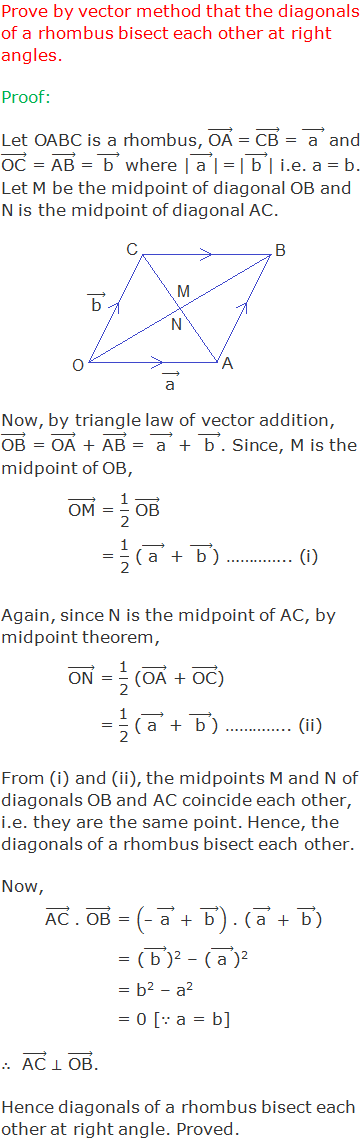# Vector GeometryVector Geometry: We can study different properties and relations relating to geometry with the help of vectors. Such a study is known as the Vector Geometry.

********************

10 Math Problems officially announces the release of Quick Math Solver, an Android App on the Google Play Store for students around the world.

********************

Here are 12 geometrical theorems and their proofs by vector method.

## Theorem 1: Midpoint Formula## Theorem 2: Section Formula for Internal Division## Theorem 3: Section Formula: External Division## Theorem 4: Centroid Formula## Theorem 5:## Theorem 6:## Theorem 7:## Theorem 8:## Theorem 9:## Theorem 10:## Theorem 11:## Theorem 12:### Do you have any questions regarding the vector geometry?

You can ask your questions or problems here, in the comment section below.